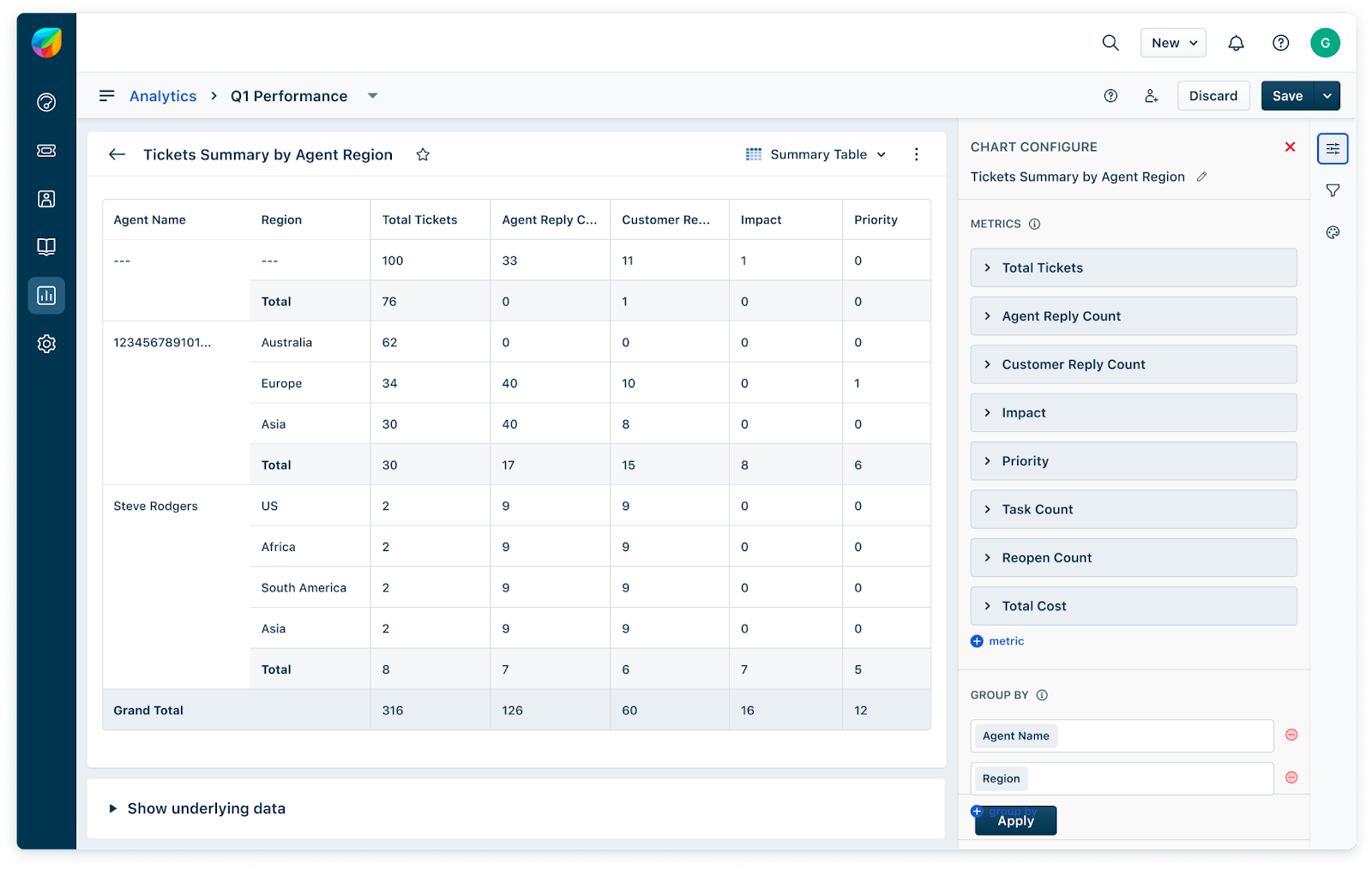Sub Totals and Grand Totals help you visualise multiple levels of summary data at one go. Sub Totals is calculated as an aggregation of a specific set of metric data at an each individual grouping where as Grand Total is calculation on all the detailed data of that metric.

A Grand Total is a total of all calculations performed on a metric. A Sub Total is a total of a specific subset of metric data, totaled at the groups selected.

There are two ways this feature can be utilised:

1. The Summary table will allow users to apply totals on rows, and

2. The Matrix table will allow users to apply totals on both rows and columns.